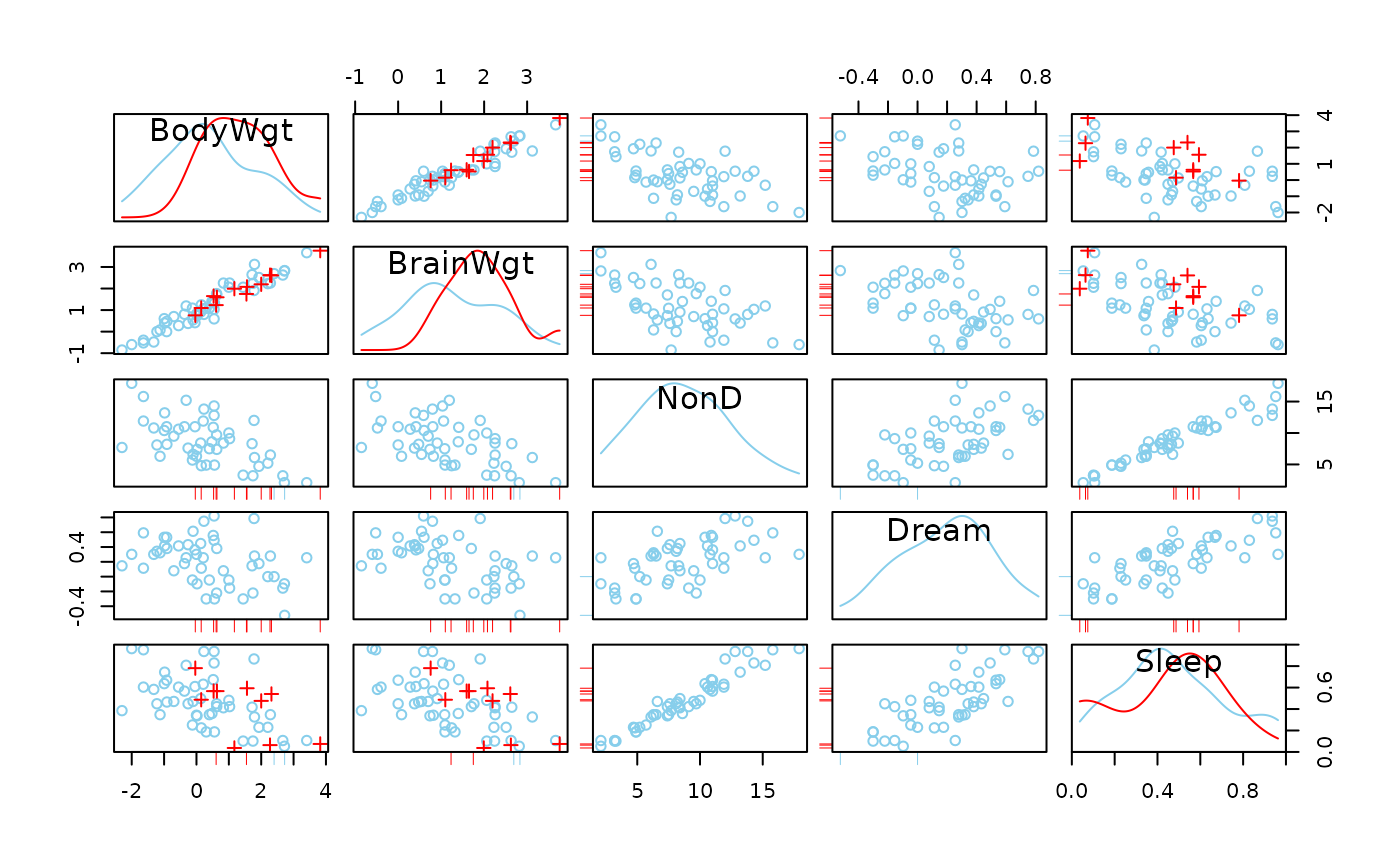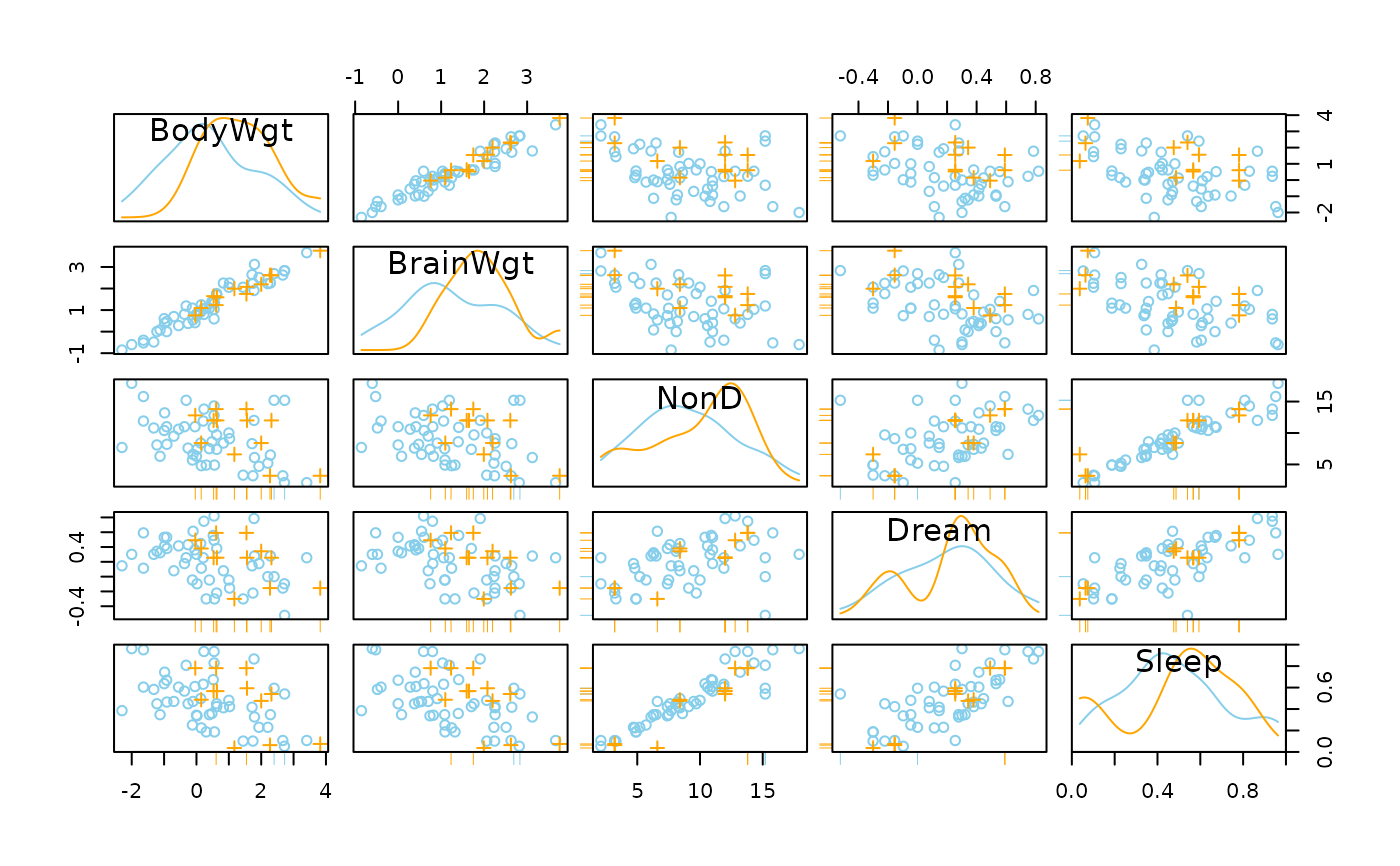Scatterplot matrix in which observations with missing/imputed values in certain variables are highlighted.

scattmatrixMiss(
x,
delimiter = NULL,
highlight = NULL,
selection = c("any", "all"),
plotvars = NULL,
col = c("skyblue", "red", "orange"),
alpha = NULL,
pch = c(1, 3),
lty = par("lty"),
diagonal = c("density", "none"),
interactive = TRUE,
...
)

## Arguments

x

a matrix or data.frame.

delimiter

a character-vector to distinguish between variables and imputation-indices for imputed variables (therefore, x needs to have colnames()). If given, it is used to determine the corresponding imputation-index for any imputed variable (a logical-vector indicating which values of the variable have been imputed). If such imputation-indices are found, they are used for highlighting and the colors are adjusted according to the given colors for imputed variables (see col).

highlight

a vector giving the variables to be used for highlighting. If NULL (the default), all variables are used for highlighting.

selection

the selection method for highlighting missing/imputed values in multiple highlight variables. Possible values are "any" (highlighting of missing/imputed values in any of the highlight variables) and "all" (highlighting of missing/imputed values in all of the highlight variables).

plotvars

a vector giving the variables to be plotted. If NULL (the default), all variables are plotted.

col

a vector of length three giving the colors to be used in the plot. The second/third color will be used for highlighting missing/imputed values.

alpha

a numeric value between 0 and 1 giving the level of transparency of the colors, or NULL. This can be used to prevent overplotting.

pch

a vector of length two giving the plot characters. The second plot character will be used for the highlighted observations.

lty

a vector of length two giving the line types for the density plots in the diagonal panels (if diagonal="density"). The second line type is used for the highlighted observations. If a single value is supplied, it is used for both non-highlighted and highlighted observations.

diagonal

a character string specifying the plot to be drawn in the diagonal panels. Possible values are "density" (density plots for non-highlighted and highlighted observations) and "none".

interactive

a logical indicating whether the variables to be used for highlighting can be selected interactively (see ‘Details’).

...

for scattmatrixMiss, further arguments and graphical parameters to be passed to pairsVIM(). par("oma") will be set appropriately unless supplied (see graphics::par()). For TKRscattmatrixMiss, further arguments to be passed to scattmatrixMiss.

## Details

scattmatrixMiss uses pairsVIM() with a panel function that allows highlighting of missing/imputed values.

If interactive=TRUE, the variables to be used for highlighting can be selected interactively. Observations with missing/imputed values in any or in all of the selected variables are highlighted (as determined by selection). A variable can be added to the selection by clicking in a diagonal panel. If a variable is already selected, clicking on the corresponding diagonal panel removes it from the selection. Clicking anywhere else quits the interactive session.

The graphical parameter oma will be set unless supplied as an argument.

TKRscattmatrixMiss behaves like scattmatrixMiss, but uses tkrplot to embed the plot in a Tcl/Tk window. This is useful if the number of variables is large, because scrollbars allow to move from one part of the plot to another.

## Note

Some of the argument names and positions have changed with version 1.3 due to a re-implementation and for more consistency with other plot functions in VIM. For back compatibility, the argument colcomb can still be supplied to ...{} and is handled correctly. Nevertheless, it is deprecated and no longer documented. Use highlight instead. The arguments smooth, reg.line and legend.plot are no longer used and ignored if supplied.

pairsVIM(), marginmatrix()

Other plotting functions: aggr(), barMiss(), histMiss(), marginmatrix(), marginplot(), matrixplot(), mosaicMiss(), pairsVIM(), parcoordMiss(), pbox(), scattJitt(), scattMiss(), spineMiss()

## Author

Andreas Alfons, Matthias Templ, modifications by Bernd Prantner

## Examples


data(sleep, package = "VIM")
## for missing values
x <- sleep[, 1:5]
x[,c(1,2,4)] <- log10(x[,c(1,2,4)])
scattmatrixMiss(x, highlight = "Dream")
#> Warning: variable 'Dream' contains infinite values## for imputed values
x_imp <- kNN(sleep[, 1:5])
x_imp[,c(1,2,4)] <- log10(x_imp[,c(1,2,4)])
scattmatrixMiss(x_imp, delimiter = "_imp", highlight = "Dream")
#> Warning: variable 'Dream' contains infinite values